Home > A2C > Chapter 9 > Lesson 9.1.2 > Problem9-46

9-46.
1. For each equation, find two solutions 0° ≤ θ < 360°, which make the equation true. You should not need a calculator. Homework Help ✎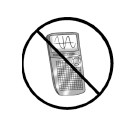1.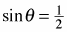2. tanθ =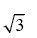3. cosθ =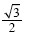4.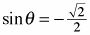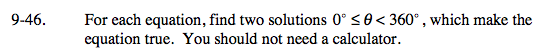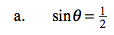30° and 150°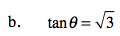60° and ?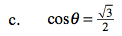30° and ?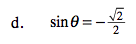225° and ?